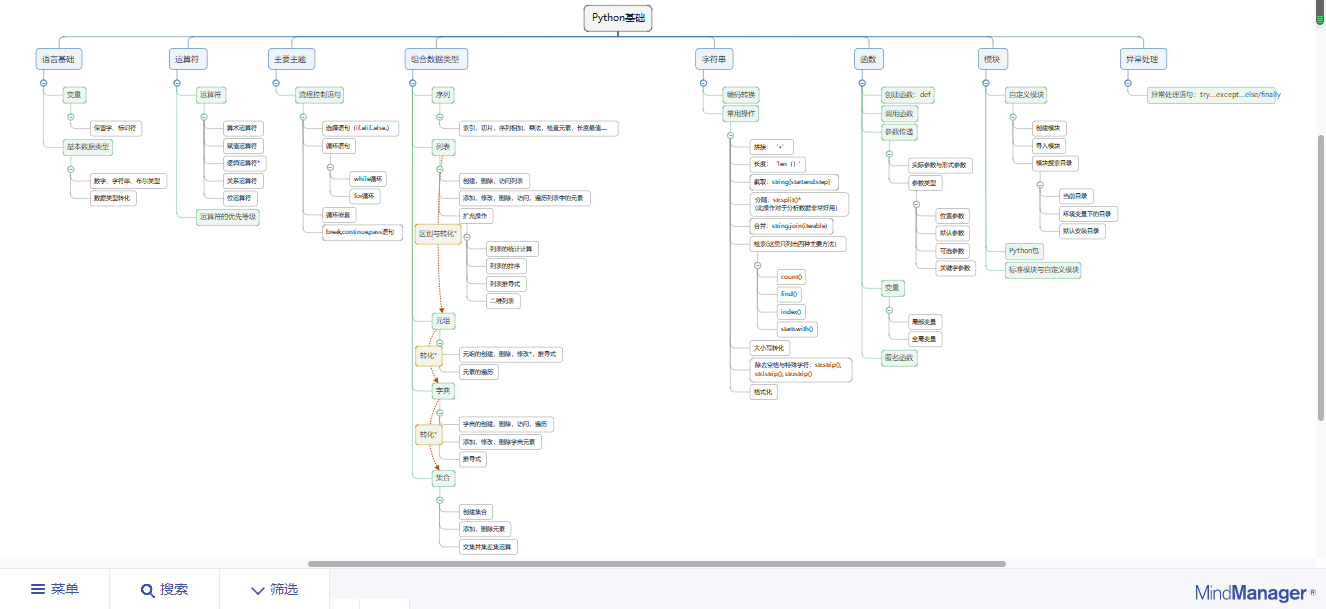# 从小白开始，洞察Python之妙

——初学者的回忆录

### Python基础知识思维导图：## 回顾学习进程：

1.输入超过五位数的整数，输出数字+万
（初识Python）

`X = int(input("请输入一个超过五位数的整数"))  #将输入的X转换为整数类型X_A = int( X / 10000 )#将输入的X除以10000X_K = int( X / 1000 ) #将输入的X除以1000print(X_A,"万")   #赋予单位万print(X_K,"K")#赋予单位K`

2.计算学分绩点
（小试身手）

`score = 0credit = 0suject_number = 0GPA = 0Average_GPA = 0a = 0while True:score =input("请输入该课程的分数：")credit = int(input("请输入该课程的学分："))if score == "stop":breakif score == "优秀":GPA =4.5suject_number = suject_number + 1a = GPA*creditprint(GPA)if score == "良好":GPA =3.5suject_number = suject_number + 1a = a+GPA*creditprint(GPA)if score == "中等":GPA =2.5suject_number = suject_number +1a = a+GPA*creditprint(GPA)if score == "及格":GPA =1.5suject_number =suject_number +1a = a+GPA*creditprint(GPA)if score == "不及格":GPA = 0suject_number =suject_number +1a = a+GPA*creditprint(GPA)print(a/suject_number)`

3.打印九九乘法表
（举一反三）

`for i in range(1,10):for x in range(1,10):print(str(i)+"*"+str(x)+"="+str(i*x),end="  ")`

4.寻找2017年1月到3月的周一/二/…/日
（再接再厉）

`a=[]b=[]for x in range(20170101,20170132): #在for in 遍历中用内置函数range创建列表a.append(x)#将列表放入a中for y in range(20170201,20170229):a.append(y)   #同上for z in range(20170301,20170332):a.append(z)#同上b=a[ : :6] #用步数做成切片print(b)`

5.输入数字，输出对应的星期数
(学而不厌）

`w="星期一 星期二 星期三 星期四 星期五 星期六 星期天"f=w.split(" ")a=eval(input('请输入表示星期的而数字:'))print(f[a-1])`

6.字符串练习
(训练日常）

`s = 'abcABCabc's.lower() #小写s.upper()#大写`

`a = 'a b c 123,456'a.split(" ")#分隔`

`v = '1234556'v.count('5')#计数`

`f = '\$\$50%%'f.strip('\$%')#删除`

`j = 'abcde''@'.join(j)#增加`

7.算法练习
（训练日常）

`isinstance(12j, float) + 12   #isinstance判断，返回布尔值`

`int('22', 16)#22的16进制`

`int(abs(4-3j))  #4-3j 的绝对值（模）`

`int( 50 ** .5 ) #50开根，输出整数部分`

`(not 3) + 5  #not 3 输出布尔值0`

### 希望我的分享能让大家更熟悉Python这门语言。

#### 最新留言

[***.215.79.68]2022年07月04日 20时55分21秒

## 关于作者喝酒易醉，品茶养心，人生如梦，品茶悟道，何以解忧？唯有杜康！
-- 愿君每日到此一游！